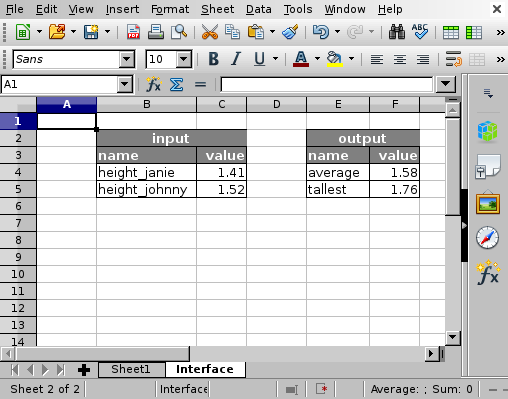# Usage¶

Suppose we have the following table with heights of family members.Example table.

Since Janie and Johnny have not reached full height, we might want to export their heights as input variables. Suppose we are interested in the average height and the tallest person in this family. This information goes to the “Interface” sheet.Example interface.

In this sheet we put the input variables in column `B` and references to the values in column `C`. The value of `C4` is `=\$Sheet1.C5` and that of `C5` is `=\$Sheet1.C6`.

Likewise, the output variables are put in column `E` and references to the values in column `F`. The value of `F4` is `=\$Sheet1.C7` and that of `F5` is `=\$Sheet1.C9`.

## Command line interface¶

With the command line interface, the input and output table can be read.

```\$ spreadscript read_input data/test.ods
{"height_janie": 1.41, "height_johnny": 1.52}

To manipulate the input, use the `process` subcommand:
```\$ spreadscript process data/test.ods  '{"height_johnny": 1.56}'